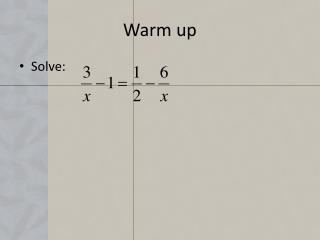DownloadDownload PresentationWarm up

# Warm up

Download Presentation## Warm up

- - - - - - - - - - - - - - - - - - - - - - - - - - - E N D - - - - - - - - - - - - - - - - - - - - - - - - - - -
##### Presentation Transcript

1. Warm up • Solve:

2. Lesson 2-2 Applications of Algebra Objective: To use algebra to solve word problems

3. Problem Solving Steps • 1. Read the problem carefully • 2. Define the variable • 3. Write the equation • 4. Solve the problem • 5. Check you work!

4. Single Variable Problems • Prices & Discounts • If you pay \$50 for a pair of shoes after receiving a 20% discount, what was the price of the shoes before the discount? • Let s – price of shoes before discount • Discount = .20s • s - .20s = 50

5. Prices and Discounts • If you pay \$75 for a new phone after receiving a discount, and the original price was \$125. How much discount did you receive?

6. Coin Problems • Carrie has 40 more nickels than Joan has dimes. They both have the same amount of money. How many coins does each girl have? • Let x = the number of coins that Joan has. • 5(40 + x) = 10x • 200 + 5x = 10x • 200 = 5x • 40 = x

7. Coin Problems • Karl has some nickels and pennies totaling \$1.80. He has 4 fewer pennies than three times the number of nickels. How many of each does he have?

8. Simple Interest • Interest (I) = Principal(P) x rate(R) x time(t) • Principal= amount borrowed or invested • Total amount owed: S = P + I = P + Prt

9. Simple Interest • A part of \$10,000 was borrowed at 3% simple annual interest and the remainder at 5%. If the total amount of interest due after 3 years is \$1275, how much was borrowed at each rate? 1275 = 0.09s + 0.15(10000-s)

10. Simple Interest • A part of \$25,000 was borrowed at 7% simple annual interest and the remainder at 4%. If the total amount of interest due after 4 years is \$5000, how much was borrowed at each rate?

11. Distance Problems (Uniform Motion) • Distance = Rate x Time (d=rt) • Are the distances equal? • Do they add together to a total?

12. Distance Problems (Uniform Motion) • Mary & Michael leave school traveling in opposite directions. Michael is walking and Mary is biking, averaging 6 km/h more than Michael. If they are 18 km apart after 1.5 h, what is the rate of each?

13. Distance Problems (Uniform Motion) • Andrew begins biking south at 20 km/h at noon. Justin leaves from the same point 15 min. later to catch up with him. If Justin bikes at 24 km./h, how long will it take him to catch up to Andrew?

14. Warm up • Erin drove her car to the garage at 48 km/ h and then walked back home at 8 km/h. The drive took 10 min less than the walk home. How far did Erin walk and for how long?

15. Mixture Problems • A grocer makes a natural breakfast cereal by mixing oat cereal costing \$2 per kilogram with dried fruits costing \$9 per kilogram. How many kilograms of each are needed to make 60 kg of cereal costing \$3.75 per kilogram?

16. Mixture Problems • How many liters of water must be added to 20L of a 24% acid solution to make a solution that is 8% acid?

17. Work Problems • Involves 2 or more people or machines completing a task. • The rate of work per unit of time is usually a fraction. (If it takes 3 hour for it to complete the job the rate is 1/3 of a job per hour) • Rate = job • Work done = (Rate)(Time)

18. Work Problems • One printing press can finish a job in 8 h. The same job would take a second press 12 h. How long would it take both presses together?

19. Work Problems • A mail handler needs 3 h to sort an average day’s mail, but with an assistant it takes 2h. How long would it take the assistant to sort the mail working alone?